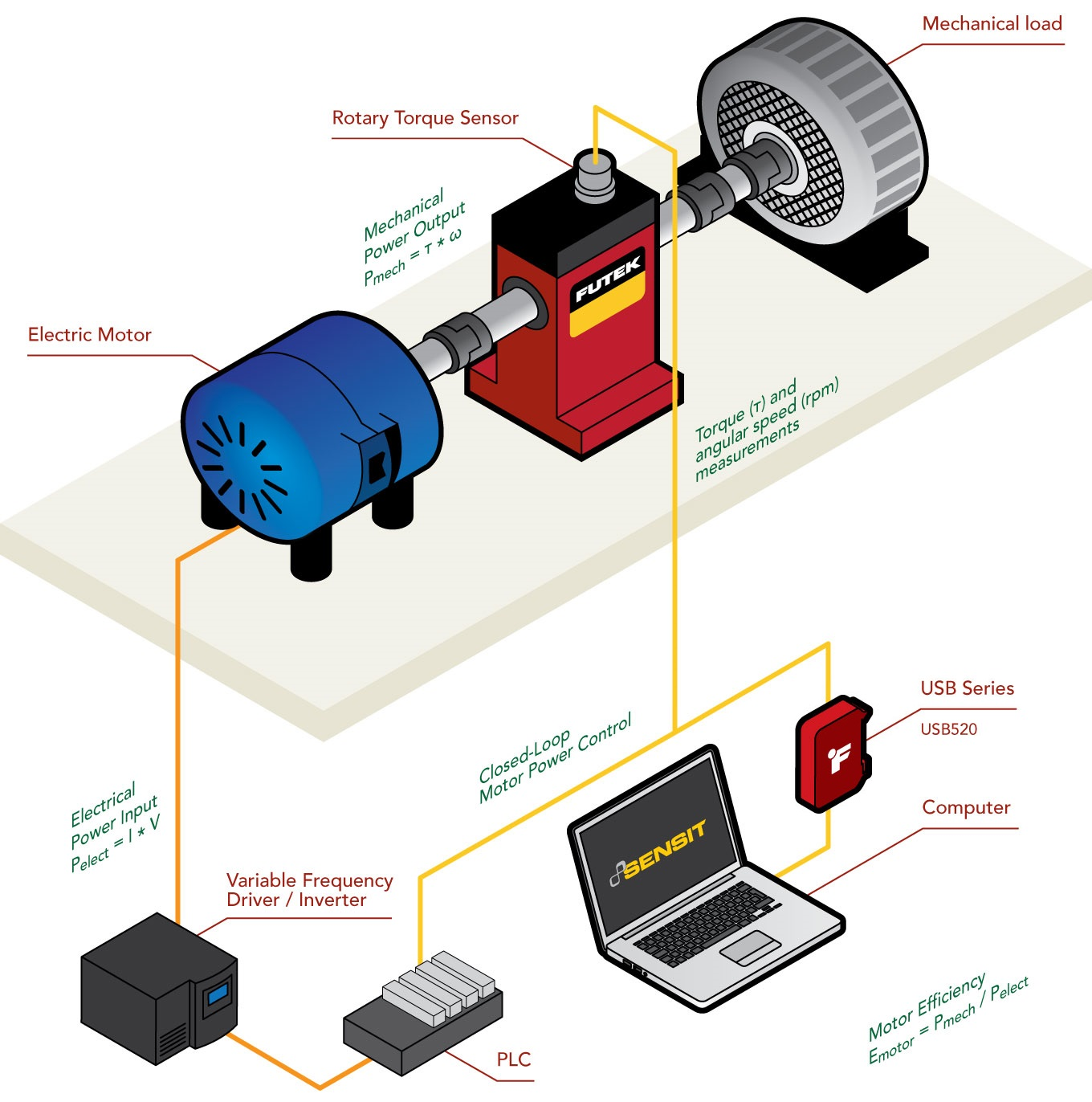Mode Layout
Theme color
Background Color:
Reset

# Electrical Motor Efficiency

.

### Basic principles of electric motor efficiency :

Electric motors are electromechanical machines that convert electrical energy into mechanical energy through a rotating shaft. Despite differences in size and type, most electric motors operate on the same basic principle: an electric current flowing through a coil of wire in a magnetic field (stator) creates a force that rotates the coil (rotor), thus creating torque (rotating shaft).

In this scenario, the efficiency of the motor is a ratio of how well the motor converts electrical energy into mechanical energy and is calculated by dividing the electrical power supplied to the motor by the mechanical power generated by the motor. The mechanical power output is then calculated based on the torque and angular velocity, and the electrical power input is calculated based on the voltage and current supplied to the motor. The mechanical power output is always less than the electrical power input because energy is lost during the conversion process in various forms, such as heat, friction, ohmic losses and noise.

.

### Electric Motor Output Power :

As described above, electric motors convert electrical energy into mechanical work. Two important variables determine the mechanical capabilities of a motor: angular velocity and torque. The mechanical power output of the motor could be calculated using the following formula:

Pmec = Pout = τ * ω

While the electrical power consumed by the motor is defined by the equation :

Pelec = Pin = I * V

It is thus possible to calculate the efficiency of the motor by dividing the mechanical output power by the electrical input power,
which gives us :

Motor efficiency = Pmec / Pelec = Pout / Pin

If the motor has an efficiency of 100%, all the electrical power is converted into mechanical energy (Pin = Pout). However, such motors do not exist. Even small, precision-made industrial motors have a maximum efficiency of 50-60%.

.

### How to measure the power of a motor ?

As mentioned above, determining the power output of a motor requires the simultaneous measurement of two variables: torque and angular velocity. For accurate and precise measurement of torque and angular velocity, we recommend the use of a rotary torque transducer with encoder in conjunction with the USB520 universal signal conditioner.

..

### How it works :

1. Our rotary torque sensors with encoder combined with the USB520 form an effective tool for motor power testing/auditing, feedback control, and electric motor torque monitoring and performance analysis.

2. When the motor is running, the sensor measures the torque produced by the motor in response to the load generated by the mechanical load.

3. We have torque sensors with integrated encoders. These allow us to measure the angle to speed ratio (RPM) produced during this test.

4. The USB520 is ideal for signal conditioning systems that require measurement of rotary torque and encoder readings, such as angle and speed. Its compact and rugged design also makes the USB520 suitable for this type of industrial application.

5. Applying FUTEK's SENSIT™ test and measurement software to this test platform will allow the operator to perform live graphing and record results.

6. The output of the USB520 can be integrated with a PLC / VFD solution for closed loop motor power control.

.

### Products Used :

• TRS/TRH/TRD series - Rotary torque transducers with built-in encoder.
• USB520 series - USB solution for torque and angle/speed measurement applications.